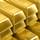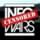It seems that you're using an outdated browser. Some things may not work as they should (or don't work at all).
We suggest you upgrade newer and better browser like: Chrome, Firefox, Internet Explorer or Opera

×
In other words, chaces of drawing an L or 2 are just below 65%.With 6F 2L, chances of F F F are

6C3 / 8C3 = 20 / 56 = 35% (rounded)
I'm using a binary method of actual combinations that exist. So i'll break it down.

In order to calculate every combination in 2 in 8 you do 8*7=56; That retains the order though. To remove the order we divide 1*2. So 56/2=28. But we are more interested in the draw of 3. Were there 3 L's it would be 8*7*6 / 1*2*3, etc.

Figuring the drawn cards it's calculated a little differently; Drawing 3 cards, 5 will be left, so we just change how many L's are left to get the odds. Also i don't care about the order of where the L is on the right 3, only if it's there.

5*4 / 2 = 10 (2 L's, since neither are drawn)
5 / 1 = 5 (1 L so 1 L must have been drawn)
1 = 1 (There's only 1 state with both L's drawn)

This adds to 10; Thus the 10/16 is the odds of 3F's. If my logic in calculating this is wrong, point it out and I'll fix it.ZFR: In other words, chaces of drawing an L or 2 are just below 65%.
Hmmm... i thought the order wasn't relevant... but maybe it was.

10
5*3=15
1*3=3

10+15+3 = 28... The full combinations present. alright...

So using these numbers instead, 18/28 = 64.28% of drawing an L...

Okay, so now i know where my math was going wrong, and why it was wrong.With 6F 2L, chances of F F F are

6C3 / 8C3 = 20 / 56 = 35% (rounded)rtcvb32: I'm using a binary method of actual combinations that exist. So i'll break it down.

In order to calculate every combination in 2 in 8 you do 8*7=56; That retains the order though. To remove the order we divide 1*2. So 56/2=28. But we are more interested in the draw of 3. Were there 3 L's it would be 8*7*6 / 1*2*3, etc.
Up to this point correct. There are 56 orderless combinations.

There are 6 F cards. How many 3-card orderledd combinations? 6x5x4/3x2x1 =20.

So out of 56 combinations 20 involve FFF.

Probability is 20/56.

Not sure what you're doing next. Can you explain.

OR

Probability of drawing first F is 6/8
Second is 5/7 since 7 cards are left with 5 F.
Third is 4/6.

Probability of first 3 being f is 6/8 x 5/7 x 4/6 = 20/56.ZFR: Up to this point correct. There are 56 orderless combinations.
Ordered actually, you could have L1 and L2 and that combinations lets you know in what order they are. There's 28 orderless combinations. Still if you're doing both of them ordered it doesn't affect the math.ZFR: There are 6 F cards. How many 3-card orderless combinations? 6x5x4 / 3x2x1 =20.

So out of 56 combinations 20 involve FFF.
or 28 & 10 (divided by half), which we both agree on. The 20 throws me off but i can see how it goes together.ZFR: Not sure what you're doing next. Can you explain.
Well i WAS just doing the raw combinations using 5 remaining cards and ignoring the order of the L's, but i see that was wrong. Thus x3 fixes those (and adding to 28 confirms it).ZFR: OR

Probability of drawing first F is 6/8
Second is 5/7 since 7 cards are left with 5 F.
Third is 4/6.

Probability of first 3 being f is 6/8 x 5/7 x 4/6 = 20/56.
With programming, blundering through sometimes until you get the proper answer is how it works :P Been developing a method of figuring out these combinations to do data compression.

I suppose my lack of Algebra makes me feel like a dunce here.
If you still want to make sure write it out manualy.
1 2 3 4 5 6 fascist
7 8 liberal

Possible 3-card combinations
123
124
125
126
127
128
134
135
136
.... etc (56 in total)

See how many contain 7 or 8? You will find that 36 of them do.ZFR: If you still want to make sure write it out manualy.

<snip>

See how many contain 7 or 8? You will find that 36 of them do.
I'd brute force it and then use regex to have it show up. Which is easy enough to do. But you don't want to do 1-8, you want to do 1 & 2 or 0 & 1.

Hmmm might as well, i'll try brute forcing it and submit a picture of the results.ZFR: Up to this point correct. There are 56 orderless combinations.rtcvb32: Ordered actually,
Orderless. Ordered is where order matters.

Manually writing ordered ones means 123 132 213 231 312 321 are different combinations. So there are more ordered than orderless. Since for orderless all the 6 above would just be 1 combination.

So there 8x7x6/3x2x1 =56 orderless combinations. Mathematically can be written as nCr or using the binomial coefficient.

And 8x7x6 = 336 ordered. These are called permutations and mathematically written as nPr.ZFR: Orderless. Ordered is where order matters.
But 56 is ordered combinations. at least with 2 & 8.

Regardless... Regex brute force :P
Attachments:
brute.png (8 Kb)ZFR: If you still want to make sure write it out manualy.

<snip>

See how many contain 7 or 8? You will find that 36 of them do.rtcvb32: I'd brute force it and then use regex to have it show up. Which is easy enough to do. But you don't want to do 1-8, you want to do 1 & 2 or 0 & 1.

Hmmm might as well, i'll try brute forcing it and submit a picture of the results.
I "want" to do 1 to 8 so each card has a different number and you can differentiate one fascist card from another.ZFR: Orderless. Ordered is where order matters.rtcvb32: But 56 is ordered combinations. at least with 2 & 8.

Regardless... Regex brute force :P
But Im talking about 3 and 8. 3 cards drawn out of 8.rtcvb32: But 56 is ordered combinations. at least with 2 & 8.

Regardless... Regex brute force :PZFR: But Im talking about 3 and 8. 3 cards drawn out of 8.
And i'm talking the number of bits/cards that are L/F and the combinations/states it could be in. Building on that combination number. By removing 3 cards/bits to 5, i got the original 10/5/1 values (should've been 10/15/3).

On the right side (of brute.png) note the 3 digits in the last regex are the only ones that are highlighted, thus the 3 cards drawn in this case. Shouldn't be hard to confirm.

Still we're in agreement where we should be.rtcvb32: Still we're in agreement where we should be.
Yes I can see now how you approached it.ZFR: Yes I can see now how you approached it.
Regardless, thanks for directly (or indirectly) helping me with my math in this area, and figuring out where my mistakes were in trying to do these calculations. It's more or less why my odds have probably been off on a number of my posts when trying to rely on this method; Since mixing the before & after 3 cards was throwing me totally off and i couldn't explain it.

But back to the game.
So instead of looking at combinations of drawn cards you looked at combinations of possible locations of the two liberal cards. Ok, its clear now what you meant.